Help Center
1. Help Center
2. Item Workflow
3. Chart - Tips and Tricks

Calculated Data Series

You can create an automatically calculated data series on any Bar/Line Chart. The calculated series can be a:

• Sum

• Difference

• Average

• Percent

• Percent Change

• Multiply

• Divide

How do I add a calculated data series?

Navigate to the Chart with which you want to work, then:

• Select one of the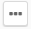icons in the top right corner of the page.

• In the resulting drop-down menu, click.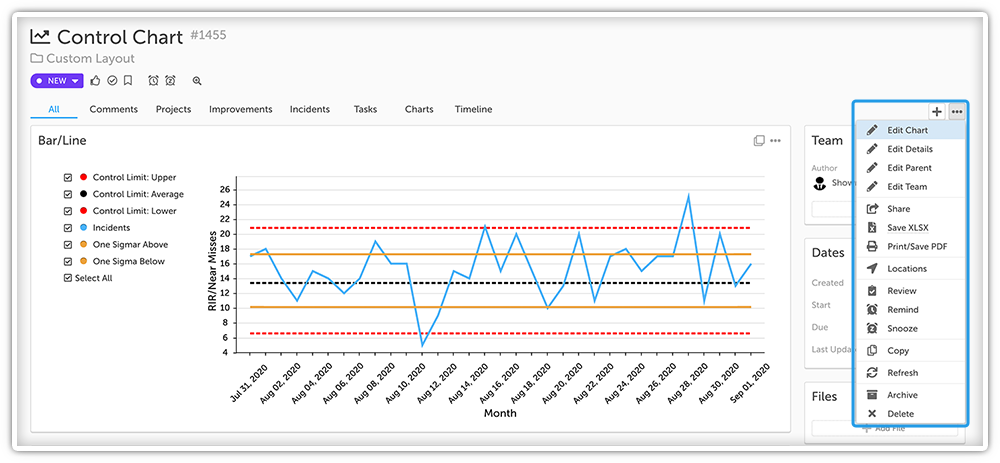or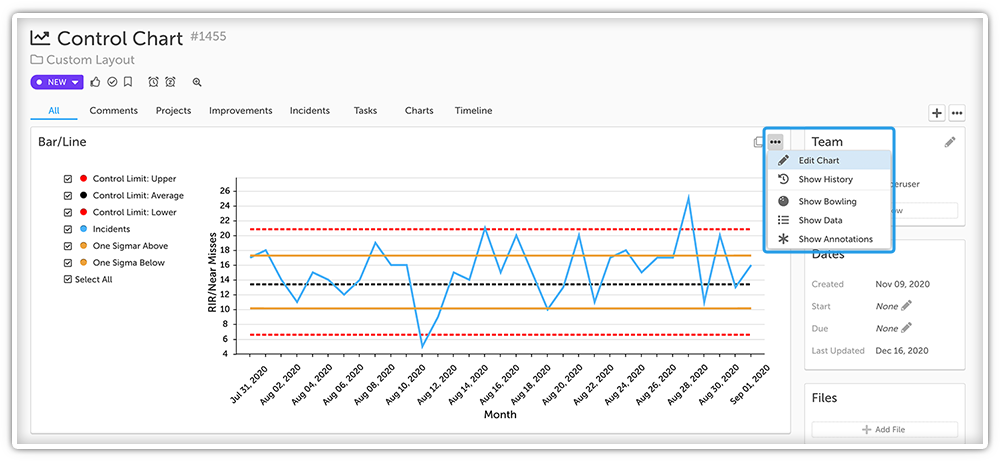In the resulting window:

• Either add a series by clicking + Add Data Series or edit an existing series by clicking its name.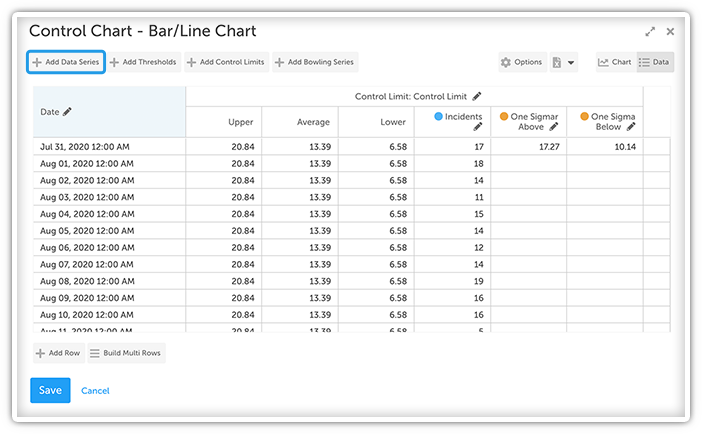• Configure the series as you normally would.

• Check the box next to "Calculated Series"

• Locate the "Operator" field under the "Calculated Series" section. In that field, choose which operator the calculated series should use. Depending on which you pick, various new fields will appear.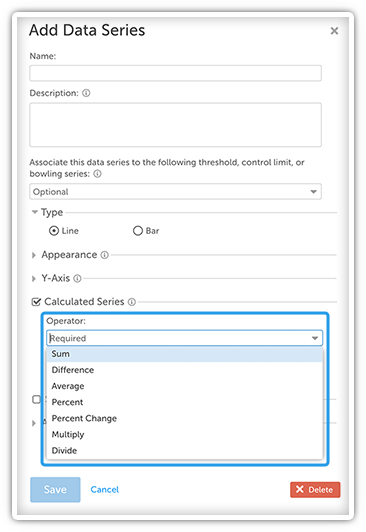NOTE: Users with appropriate chart permissions can share Calculated Series from one chart to another and use that series in a Calculated Series.

If you chose:

• Sum: Enter each series you want to be added together in the “Series” field.

• Difference: Enter the series you want to subtract from in the “Series to subtract from” field and the series you want to be subtracted in the “Series to subtract” field.

• Average: Enter each series you want to be included in the average in the “Series” field.

• Percent: Enter the series you want as the numerator in the “Numerator Series” field and the series you want as the denominator in the “Denominator Series” field.

• Percent Change: Enter the baseline series in the “Baseline or Original (% change from)” field and the current series in the “Actual or Current” field.

• Multiply: Enter each series you want to be multiplied in the “Series” field.

• Divide: Enter the series you want as the numerator in the “Numerator Series” field and the series you want as the denominator in the “Denominator Series” field.
• Select the Series you want to add to the Calculated Series.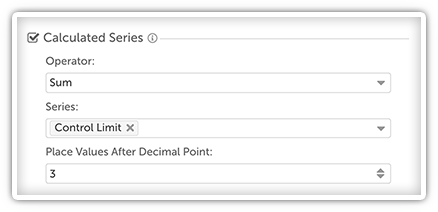• Optional: Specify the number of place values after the decimal point. For example, set the place value to 3 to calculate data to the nearest thousandth (1.234) or set the place value to 2 to calculate data to the nearest hundredth (1.23). By default, the maximum place value is 8.

• Click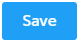to finalize your changes.

NOTE: The series’ column will be automatically filled in with the appropriate data values.